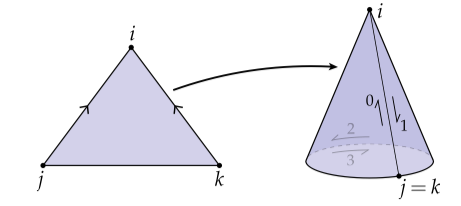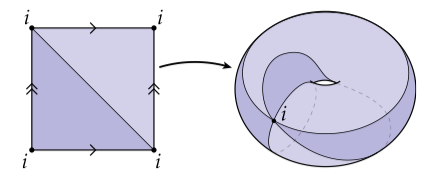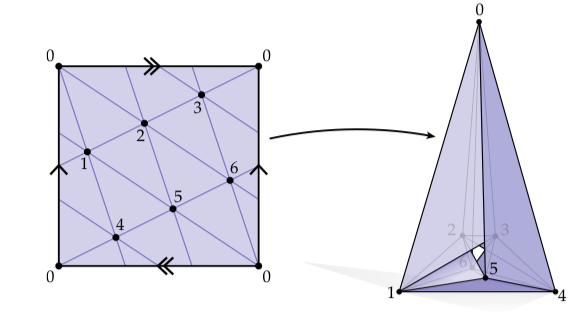DDG（离散微分几何）：组合曲面 | HyperPlane

“Everything should be made as simple as possible, but no simpler.” – Albert Einstein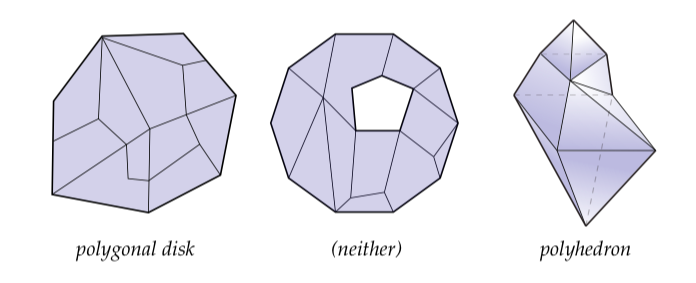## 抽象单纯复形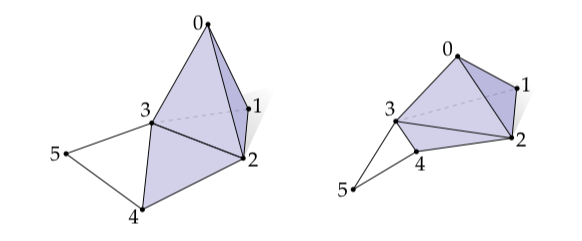图1. 一个抽象单纯复形具体描述了顶点是怎么连接的，但是没有说它们在空间的哪个位置。比如，上面的两幅图都表示同一个单纯复形，由6个顶点、10条边、5个三角形和1个四面体。


$V = \{0, 1, 2, \dots, n\}$

$\{3,4,2\}$

$\{3,5\}$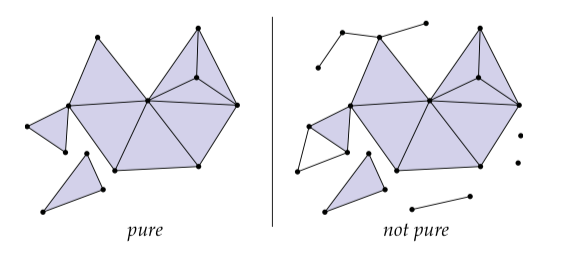## 单纯复形剖析：星、闭包和链环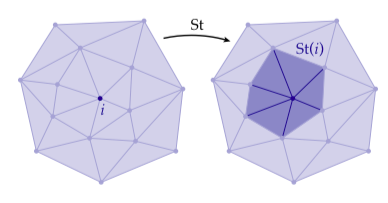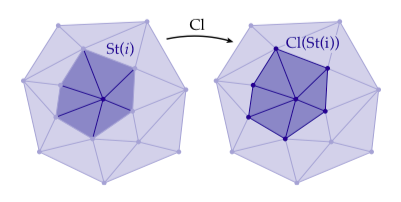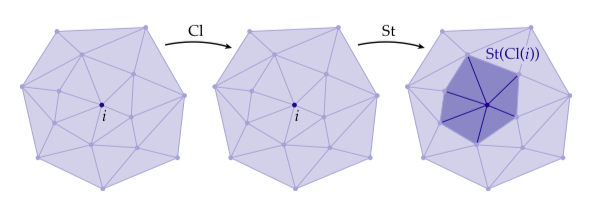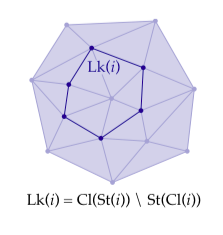• $St(S)$$\mathcal{K}$中包含$S$中任一单纯形的所有单纯形集合
• 闭包$Cl(S)$$\mathcal{K}$中包含$S$的最小（即元素最少）子复形
• $Lk(S)$等于$Cl(St(S)) \setminus St(Cl(S))$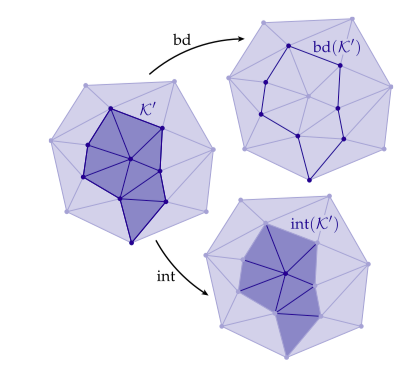### 有向单纯复形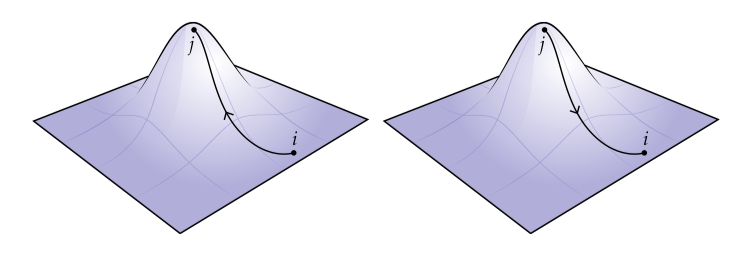$\begin{matrix} (i,j,k) & (i,k,j) \\ (j,k,i) & (j,i,k) \\ (k,i,j) & (k,j,i) \end{matrix}$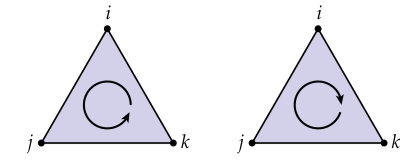$ijk \coloneqq \{(i,j,k), (j,k,i), (k,i,j)\}$

$ikj \coloneqq \{(i,k,j), (j,i,k), (k,j,i)\}$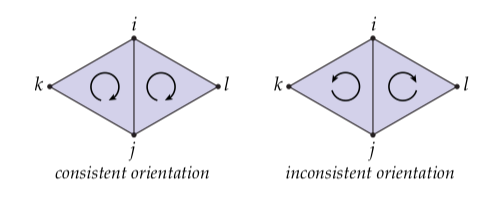## 单形曲面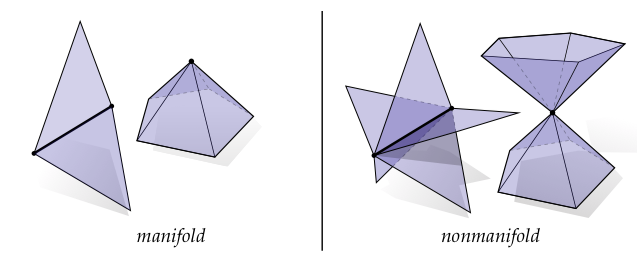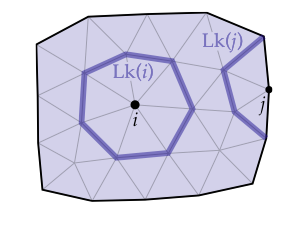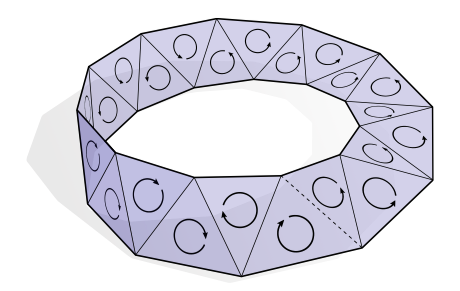$\mathbf{S}^n \coloneqq \{ x \in \mathbb{R}^{n+1} : |x|=1 \}$

## 邻接矩阵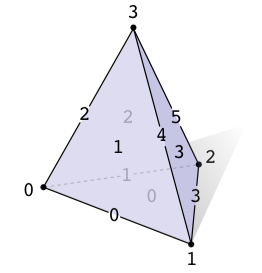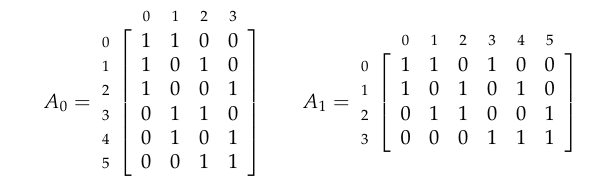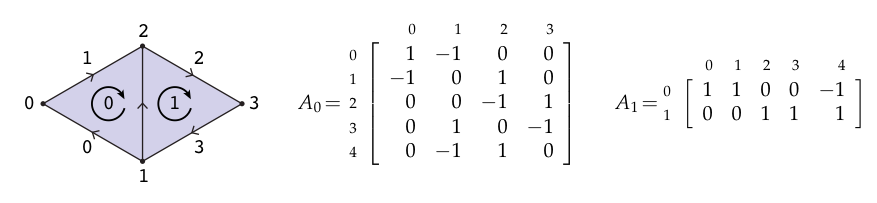## 半边网格

• 每一条边都被两个多边形包含
• 围绕一个顶点的边可以赋予一个首尾循环的顺序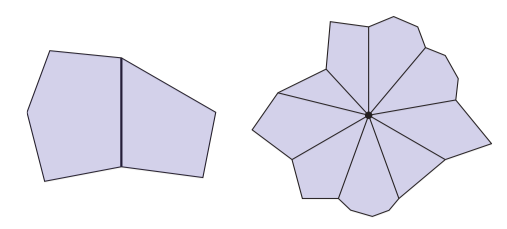$\eta(ij) = ji$

$\rho(ij) = jk , \forall ijk \in \mathcal{K}$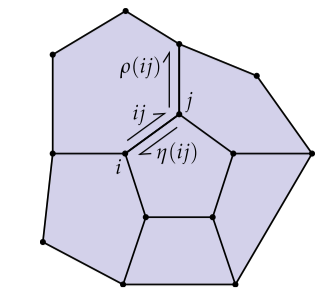• 面是$\rho$的轨道
• 边是$\eta$的轨道
• 顶点是$\rho \circ \eta$的轨道